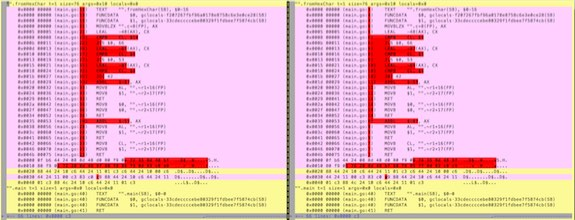# Writings - Optimizing Go's encoding/hex

Niek Sanders, June 2017

After watching Andrei Alexandrescu's Fastware, I wanted to put some of his optimization techniques into practice. This led to grepping the Go standard library for places where I could apply the "unsigned wraparoo".

The wraparoo is a strength reduction technique replacing a comparison op with an integer subtraction:

```// original code if v < '0' || v > '9' { // do something } // optimized to remove comparison vp = unsigned(v) - '0' if vp > 9 { // do something } ```

A bit of grepping in Go's source code led me to fromHexChar() in encoding/hex:

```// fromHexChar converts a hex character into its value and a success flag. func fromHexChar(c byte) (byte, bool) { switch { case '0' <= c && c <= '9': return c - '0', true case 'a' <= c && c <= 'f': return c - 'a' + 10, true case 'A' <= c && c <= 'F': return c - 'A' + 10, true } return 0, false } ```

Which I rewrote to:

```// wraparoo to cut comparisons in half func fromHexChar(c byte) (byte, bool) { cp := c - '0' if cp < 10 { return cp, true } cp = c - 'A' if cp < 6 { return cp+10, true } cp = c - 'a' if cp < 6 { return cp+10, true } return 0, false } ```

The result? Absolutely no performance benefit. In fact, the generated assembly even looks almost identical:Quite a bummer. But then I became stubborn. I spent the next hours trying tricks to speed the decode path. This let me down the rabbit hole of how Go does bound checks on array access and the fancy Bounds Check Elimination (BCE) logic that was added in Go 1.7.

It turns out that the current BCE logic is a bit limited:

```// no bounds checks with ranges for i := range mySlice { mySlice[i] = 'x' } // no bounds checks with very simple C-style loop for i := 0; i < len(mySlice); i++ { mySlice[i] = 'x' } // bound checks NOT eliminated with even modest variants for i := 0; i < len(mySlice); i += 2 { mySlice[i] = 'x' } ```

In Go 1.9 the hex.Decode function does 3 bounds checks per iteration, two for accessing `src` and one for `dst`. You can see this using the `-gcflags="-d=ssa/check_bce/debug=1"` flags at compile time.

```func Decode(dst, src []byte) (int, error) { if len(src)%2 == 1 { return 0, ErrLength } for i := 0; i < len(src)/2; i++ { a, ok := fromHexChar(src[i*2]) // <-- :( if !ok { return 0, InvalidByteError(src[i*2]) } b, ok := fromHexChar(src[i*2+1]) // <-- :( if !ok { return 0, InvalidByteError(src[i*2+1]) } dst[i] = (a << 4) | b // <-- :( } return len(src) / 2, nil } ```

I wrote variants that eliminated some (but not all) bound checks. But nothing made a real impact on hex.Decode.

I was about to give up.

But then one last idea popped in my head. Almost embarassingly simple:

```func Decode(dst, src []byte) (int, error) { if len(src)%2 == 1 { return 0, ErrLength } for i, j := 0, 0; i < len(src); i, j = i+2, j+1 { a := decodeMap[src[i]] if a == 0xFF { return 0, InvalidByteError(src[i]) } b := decodeMap[src[i+1]] if b == 0xFF { return 0, InvalidByteError(src[i+1]) } dst[j] = (a << 4) | b } return len(src) / 2, nil } ```

That decodeMap is just a simple lookup table which gets build during init. No wraparoos. No BCE shenanigans.

The improvement:

```name old time/op new time/op delta Decode/256-4 524ns ± 2% 241ns ± 1% -53.89% (p=0.000 n=10+9) Decode/1024-4 2.02µs ± 2% 0.92µs ± 1% -54.74% (p=0.000 n=10+9) Decode/4096-4 8.12µs ± 1% 3.65µs ± 1% -55.10% (p=0.000 n=9+9) Decode/16384-4 32.2µs ± 2% 14.5µs ± 2% -55.02% (p=0.000 n=9+9) ```

This led to my first Go pull request, which I'm hoping will be in Go 1.10.

>>> Writings